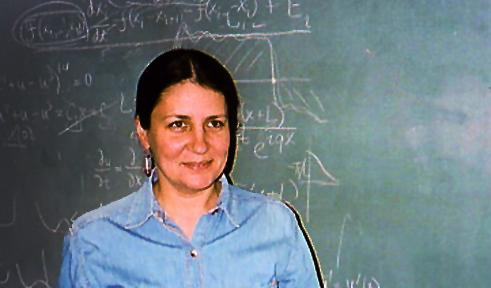# Tatiana SavinProfessor
Morton 525

## Education

Ph.D., Lomonosov Moscow State University

## Research Interests

• Applied analysis
• Analytic continuation of solutions to elliptic differential equations
• Partial differential equations
• Mathematical modeling in materials science

• Murdhy Aldawsari

• Khalid Malaikah (Ph.D., 2013)
• Lanre Akinyemi (Ph.D., 2018)

## Teaching

• Fall 2019: Math 6310 (Complex Analysis), Math 3400 (Differential Equations), Math 4970T (Mathematics Tutorial, thesis), and Math 8950 (dissertation).
• Summer 2019: Math 5910 (internship).
• Spring 2019: Math 4/5310 (Complex Variables), Math 3980T (Junior Mathematics Tutorial), Math 7400 (Topics in Differential Equations), and Math 8950 (dissertation).
• Fall 2018: Math 6310 (Complex Analysis), Math 2302 (Calculus II), Math 7400 (Topics in Differential Equations), and Math 8950 (dissertation).
• Spring 2018: Math 4/5310 (Complex Variables), Math 6930 (Independent Study), Math 6942 (Project in Mathematics Education Research), and Math 8950 (dissertation).
• Fall 2017: Math 6310 (Complex Analysis), Math 3400 (Differential Equations), Math 4310 (Complex variables), and Math 8950 (dissertation).
• Spring 2017: Math 4/5310 (Complex Variables) and Math 8950 (dissertation).
• Fall 2016: Math 3/5200 (Appl. Linear Algebra), Math 2301 (Calculus I), and Math 8950 (dissertation).
• Spring 2016: Math 3/5320 (Vector Analysis), Math 4/5310 (Complex Variables) and Math 8950 (dissertation).
• Fall 2015: Math 3/5200 (Appl. Linear Algebra), Math 6310 (Complex Analysis), Math 7300 (Topics in Analysis), and Math 8950 (dissertation).
• Spring 2015: Math 3300 (Calculus III), Math 4/5310 (Complex Variables), and Math 7300 (Topics in Analysis).
• Fall 2014: Math 7400 (Topics in Differential Equations).
• Spring 2014: Math 3300 (Calculus III), Math 4/5310 (Complex Variables), Math 4940 (Mathematics Research).
• Fall 2013: Math 2301 (Calculus I), Math 4/5400 (Advanced Differential Equations).
• Summer 2013: Math 4310 (Complex Variables) and Math 3300 (Calculus III).
• Spring 2013: Math 3/5320 (Vector Analysis), Math 4/5310 (Complex Variables), Math 8950 (dissertation).
• Fall 2012: Math 1350 (Survey of Calc.), Math 3300 (Calculus III) and Math 8950 (dissertation).
• Summer 2012: Math 895 (dissertation).
• Spring 2012: Math 670C (Complex Analysis), Math 4/570 (Complex Variables), Math 741C, Math 692.
• Winter 2012: Math 670B (Complex Analysis) and Math 266B (Calc. Appl. Biology II), Math 741B, Math 692.
• Fall 2011: Math 670A (Complex Analysis) and Math 266A (Calculus with Applications to Biology I), Math 741A.
• Summer 2011: Math 849 (Topics in Applied Mathematics) and Math 490 (Topics in Mathematics).
• Spring 2011: Math 410/510 (Matrix Theory) and Math 263A (Calculus I).
• Winter 2011: Math 340 (Differential Equations) and Math 263C (Calculus III).
• Fall 2010: Math 340 (Differential Equations) and Math 410/510 (Matrix Theory).
• Spring 2010: Math 4/570 (Complex Variables).
• Winter 2010: Math 263B (Calculus II) and Math 263C (Calculus III).
• Fall 2009: Math 4/540 (Vector Analysis) and Math 263C (Calculus III).
• Spring 2009: Math 4/510 (Matrix Theory).
• Winter 2009: Math 340 (Differential Equations) and Math 266A (Calculus with Applications to Biology I).
• Fall 2008: Math 263A (Calculus I) and Math 263B (Calculus II).
• Spring 2008: Math 4/510 (Matrix Theory).
• Winter 2008: Math 340 (Differential Equations) and Math 266A (Calculus with Applications to Biology I).
• Fall 2007: Math 340 (Differential Equations) and Math 263D (Calculus IV).
• Spring 2007: Math 4/570 (Complex Variables) and Math 266B (Calculus with Applications to Biology II).
• Winter 2007: Math 263A (Calculus I) and Math 266A (Calculus with Applications to Biology I).
• Fall 2006: Math 4/540 (Vector Analysis) and Math 690 (Complex Analysis).

## Publications

### Book Chapter

• T.V.Savina, B.Yu.Sternin and V.E.Shatalov, "Reflection Formulas and Continuation of Solutions to Boundary Value Problems", Section in the book: Boris Sternin and Victor Shatalov, Differential Equations on Complex Manifolds, Kluwer Academic Publishers, Dordeht-Boston-London, 1994.

### Preprints

• T.V.Savina, B.Yu.Sternin and V.E.Shatalov, "Notes on mother body in geophysics'" Preprint Max-Plank Institut fur Mathematik, Bonn, MPI/95-90, 23 p.